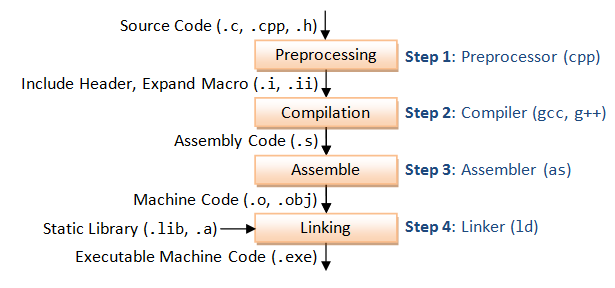🌜

# C/C++ 中的宏/MacroC/C++ 代码编译过程 - 图片来自 ntu.edu.sg

C/C++ 代码编译过程中，可通过相应参数来获取到各编译步骤中的产出，比如想看被预处理编译之后的宏，使用 gcc 使加上 -E 参数。

$gcc -E macro.c ## 宏的定义 通过 #define 指令定义一个宏。 #define NAME_OF_MACRO value 比如，以下代码定义了一个名为 BUFFER_SIZE 的宏，指代 1024 这个数字。 #define BUFFER_SIZE 1024 使用时， foo = (char *) malloc (BUFFER_SIZE); 使用预处理器编译： $ gcc -E test.c

foo = (char *) malloc (1024);

### 多行

#include <stdio.h>
#define GREETING_STR \
"hello \
world"
int main() {
printf(GREETING_STR);
return 0;
}

test.c

#include <stdio.h>
#define GREETING_STR \
"hello \
world"
int main() { printf(GREETING_STR); }

int main() {
printf("hello world");
return 0;
}

### 宏展开时的顺序

#include <stdio.h>
define GREETING_NAME "wayou"
define GREETING "hello," GREETING_NAME
int main() {
printf(GREETING);
return 0;
}

int main() {
printf("hello," "wayou");
return 0;
}

-#define GREETING_NAME "wayou"
#define GREETING "hello," GREETING_NAME
+#define GREETING_NAME "wayou"

#define BUFSIZE 1020
#define TABLESIZE BUFSIZE
#undef BUFSIZE
#define BUFSIZE 37

## Object-like 宏

Object-like 类型的宏看起来就像普通的数据对象，故名。多用于数字常量的情形下。且宏名一般使用全大写形式方便识别。像上面示例中，都是 Object-like 的。

## Function-like 宏

#define lang_init()  c_init()
lang_init()
// 编译后
c_init()

extern void foo(void);
#define foo() /* optimized inline version */
…
foo();
funcptr = foo;

#define lang_init ()    c_init()
lang_init()
// 编译后：
() c_init()()

## 宏的参数

#define min(X, Y)  ((X) < (Y) ? (X) : (Y))
x = min(a, b);          →  x = ((a) < (b) ? (a) : (b));
y = min(1, 2);          →  y = ((1) < (2) ? (1) : (2));
z = min(a + 28, *p);    →  z = ((a + 28) < (*p) ? (a + 28) : (*p));

### 入参中的括号

macro (array[x = y, x + 1])

### 入参的展开

 min (min (a, b), c)

min (((a) < (b) ? (a) : (b)), (c))

((((a) < (b) ? (a) : (b))) < (c)
? (((a) < (b) ? (a) : (b)))
: (c))

### 参数的缺省

min(, b)        → ((   ) < (b) ? (   ) : (b))
min(a, )        → ((a  ) < ( ) ? (a  ) : ( ))
min(,)          → ((   ) < ( ) ? (   ) : ( ))
min((,),)       → (((,)) < ( ) ? ((,)) : ( ))
min()      error→ macro "min" requires 2 arguments, but only 1 given
min(,,)    error→ macro "min" passed 3 arguments, but takes just 2

### 字符化/Stringizing

#define foo(x) x, "x"
foo(bar)        → bar, "x"

#define WARN_IF(EXP) \
do { if (EXP) \
fprintf (stderr, "Warning: " #EXP "\n"); } \
while (0)
WARN_IF (x == 0);
→ do { if (x == 0)
fprintf (stderr, "Warning: " "x == 0" "\n"); } while (0);

## 拼接

struct command
{
char *name;
void (*function) (void);
};
struct command commands[] =
{
{ "quit", quit_command },
{ "help", help_command },
…
};

#define COMMAND(NAME)  { #NAME, NAME ## _command }
struct command commands[] =
{
COMMAND (quit),
COMMAND (help),
…
};

## 不定参数

#define eprintf(…) fprintf (stderr, __VA_ARGS__)

eprintf ("%s:%d: ", input_file, lineno)
// 编译后：
fprintf (stderr, "%s:%d: ", input_file, lineno)

C++ 中可这么写：

#define eprintf(args…) fprintf (stderr, args)

### 不定参数与命名参数混合的情况

#define eprintf(format, …) fprintf (stderr, format, __VA_ARGS__)

## 取消和重置宏

#define FOO 4
x = FOO;        → x = 4;
#undef FOO
x = FOO;        → x = FOO;

### 两个宏相似的定义

• 类型相同，比如同为对象类型，或函数类型的宏
• 展开后各位置的符号（token）相同
• 如果是函数宏，入参相同
• 空白的不限但出现的位置相同

#define FOUR (2 + 2)
#define FOUR         (2    +    2)
#define FOUR (2 /* two */ + 2)

#define FOUR (2 + 2)
#define FOUR ( 2+2 ) // 空白位置不一样
#define FOUR (2 * 2) // 宏的内容不一样
#define FOUR(score,and,seven,years,ago) (2 + 2) // 入参不一样

## 相关资源

posted @ 2019-06-23 11:53  刘哇勇  阅读(...)  评论(...编辑  收藏